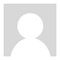Ask Remember Me?

# area of isosceles triangle given circle radii

Asked Dec 20, 2010, 02:49 PM  8 Answers
Here is a fun little geometry problem. Give it a go if you so desire.

Look at the graph. The radius of the larger circle is 2r and the radius of the smaller circle is r. Find the area of the triangle.

There are many ways to go about it. I used simialr triangles, but perhaps you have a better way.

I just left r=1 in order to graph it. But consider r to be some radius r, not 2 as I have in the graph. The area of the triangle should be expressed in terms of r alone.

Search this Questionebaines Posts: 12,127, Reputation: 1307 Expert #2 Dec 20, 2010, 03:21 PM

I get:

Similar triangles seems to be the best way.galactus Posts: 2,271, Reputation: 282 Ultra Member #3 Dec 20, 2010, 03:33 PM

Yep. That's it.

Except, I expressed it as .

Same thing.

Cool, ebaines.

This reminds me of the infinite circles inside the triangle, except it stops at 2 circles. :)harum Posts: 339, Reputation: 27 Full Member #4 Dec 20, 2010, 04:46 PM
Because FE/GD = 2, CG = FG. I.e. CH = 8r. BH = CH * tan(BCH) = CH * (2/sqrt(32)) = CH/sqrt(8) = r*sqrt(8). S = CH*BH = 16*sqrt(8)*r^2.harum Posts: 339, Reputation: 27 Full Member #5 Dec 20, 2010, 09:08 PM
Comment on harum's postOriginally Posted by harumBecause FE/GD = 2, CG = FG. I.e. CH = 8r. BH = CH * tan(BCH) = CH * (2/sqrt(32)) = CH/sqrt(8) = r*sqrt(8). S = CH*BH = 16*sqrt(8)*r^2.
Is there a way to edit a typo? 16*sqrt(2)*r^2galactus Posts: 2,271, Reputation: 282 Ultra Member #6 Dec 21, 2010, 02:03 AM

.

As long as no one else has posted afterwards, I have had no trouble editing a post.

You meantharum Posts: 339, Reputation: 27 Full Member #7 Dec 21, 2010, 02:02 PM
Comment on galactus's postOriginally Posted by galactus.

As long as no one else has posted afterwards, I have had no trouble editing a post.

You meant
Thanks! 16\sqrt{2}r^{2}galactus Posts: 2,271, Reputation: 282 Ultra Member #8 Dec 21, 2010, 02:21 PM

You have to place the tags around your LaTex code.

Math in brackets at the front and /math in brackets when closingUnknown008 Posts: 8,076, Reputation: 723 Uber Member #9 Dec 22, 2010, 12:39 PM

And it may sound weird... but the site has got several 'skins' On the one you are on, harum, you can't edit your own post. It's the 'newer skin' and I personally don't like it. You might try the skin made before that, by looking for your settings and look for something about 'Take me back to the old AMHD' or something along those lines. It's been a while I haven't been there myself.

 Question Tools Search this QuestionShow Printable VersionEmail this Page Search this Question: Advanced Search

## Check out some similar questions!

Isosceles triangle [ 3 Answers ]

Find the perimeter of an isosceles triangle if the vertex angle measures 50 degrees and the length of the base is 28 cm. help.tnx

A triangle inscribed in a circle has to be equilateral triangle for having maximum area ; how? What if it is an ellipse instead of a circle, or a conic section in general ?

Isosceles triangle [ 2 Answers ]

The area of an isosceles triangle is 30 square units.if the base measure 6 units,find the length of the altitude and the ratio of the altitude to the base?

Isosceles triangle [ 1 Answers ]

the sum of the square roots of any two sides of an isoseles triangle is equal to the square root of the remaining side. Is this statement true or false?

Altitude of an Isosceles Triangle [ 1 Answers ]

Ok, find the altitude of an isosceles triangle whose base is 10 and whose congruent sides are 9. I found how to find the alt of a right triangle but still don't really comprende. Help :D